Question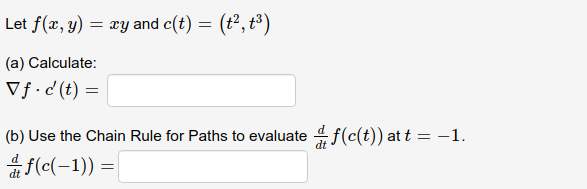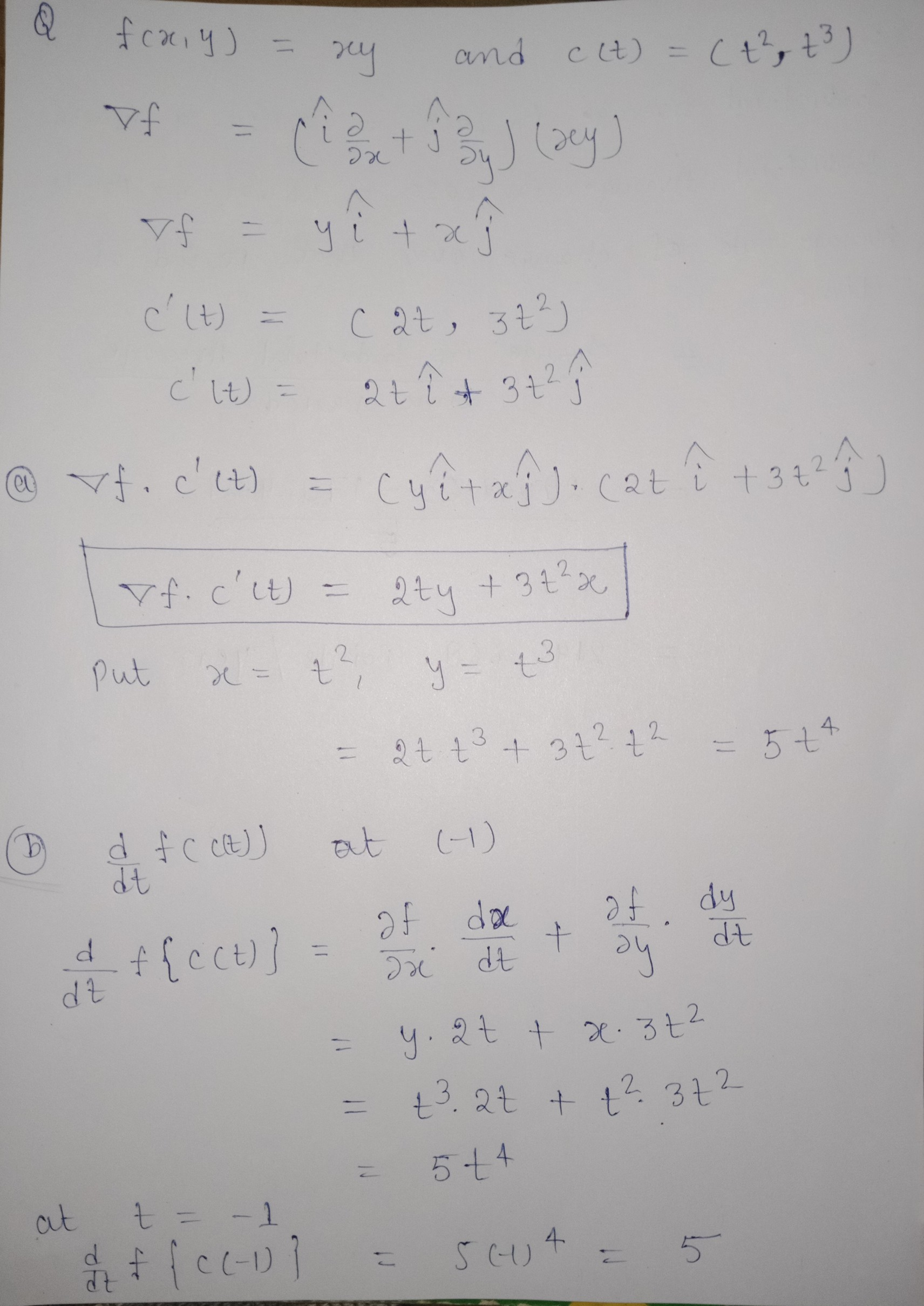if it is right than like it please give rating

#### Earn Coins

Coins can be redeemed for fabulous gifts.

Similar Homework Help Questions
• ### Exercise 3. Chain rule (15 pts) Let f(x,y,z) = xy +z-5, x = r + 2s,...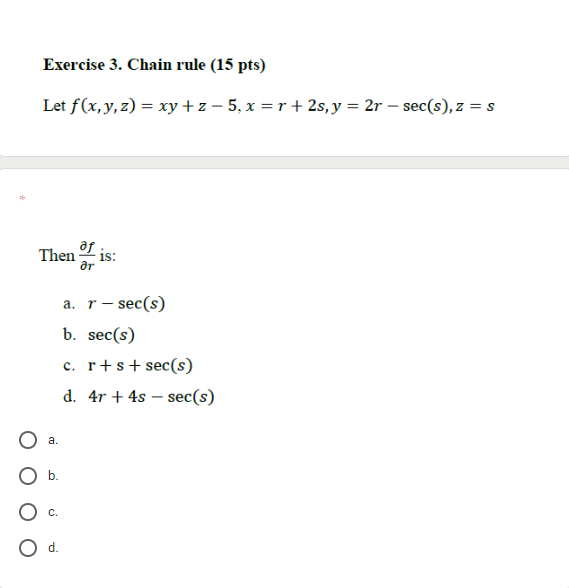Exercise 3. Chain rule (15 pts) Let f(x,y,z) = xy +z-5, x = r + 2s, y = 2r - sec(s),z=s af Then is: ar a. r - sec(s) b. sec(s) c. r+s+sec(s) d. 4r + 4s - sec(s) a. b. d.

• ### a. Use the Chain Rule to find the indicated partial derivatives. z = x4 + x2y,    x...

a. Use the Chain Rule to find the indicated partial derivatives. z = x4 + x2y,    x = s + 2t − u,    y = stu2; ∂z ∂s ∂z ∂t ∂z ∂u when s = 1, t = 2, u = 3 b. Use the Chain Rule to find the indicated partial derivatives. w = xy + yz + zx,    x = r cos(θ),    y = r sin(θ),    z = rθ; ∂w ∂r ∂w ∂θ     when r = 8, θ = pi/2 c. Use the...

• ### 4. Let f(x, y, z) = rytan'() + z sin(xy), < = wy=v²v, z = "....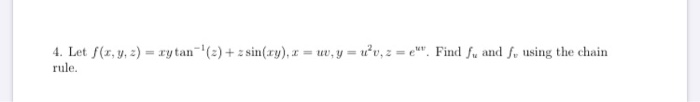4. Let f(x, y, z) = rytan'() + z sin(xy), < = wy=v²v, z = ". Find fu and , using the chain rule.

• ### Exercise 2. Directional derivative (6 pts + 9 pts) Let f(x, y, z) = xy +...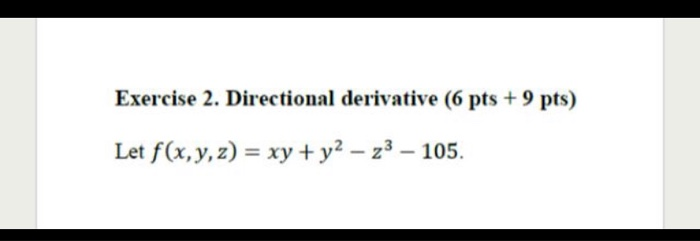Exercise 2. Directional derivative (6 pts + 9 pts) Let f(x, y, z) = xy + y2 – 23 – 105. ... touch 25% 17:12 docs.google.com 2) The direction in which f decreases most rapidly at A(0,1,1) is: a. e. None of the above a. b. C. Exercise 3. Chain rule (15 pts) Let f(x,y,z) = xy +z-5,x=r+2s,y = 2r - sec(s),z=s

• ### - bram , July It, 2014 I (a) If z=f(x,y) welt u=x²-yz and U=xy find the...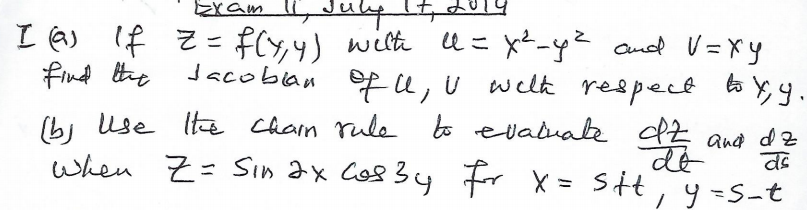- bram , July It, 2014 I (a) If z=f(x,y) welt u=x²-yz and U=xy find the Tacoblan of le, U with respect to yy. (b) Use the chain rule to evaluate az and dz When Z= sin ax cosby for x=sity-s-t व

• ### 9) Given z = x² + xy?, use the Chain Rule to find where x =...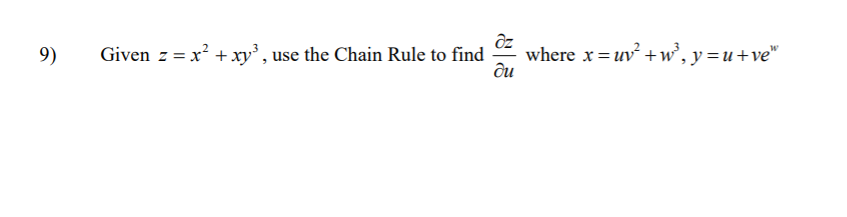9) Given z = x² + xy?, use the Chain Rule to find where x = uv? +wº, y=u+ve" ди

• ### 4. Consider the functions f : R2 R2 and g R2 R2 given by f(x, y) (x, xy) and g(x, y)-(x2 + y, x +...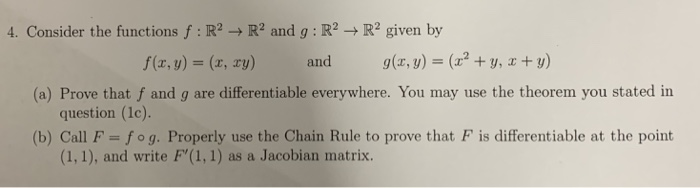4. Consider the functions f : R2 R2 and g R2 R2 given by f(x, y) (x, xy) and g(x, y)-(x2 + y, x + y) (a) Prove that f and g are differentiable everywhere. You may use the theorem you stated in (b) Call F-fog. Properly use the Chain Rule to prove that F is differentiable at the point question (1c). (1,1), and write F'(1, 1) as a Jacobian matrix. 4. Consider the functions f : R2 R2 and...

• ### please help with these questions 7. Find fryzx, for f(x, y, z) = 3 + 2?x...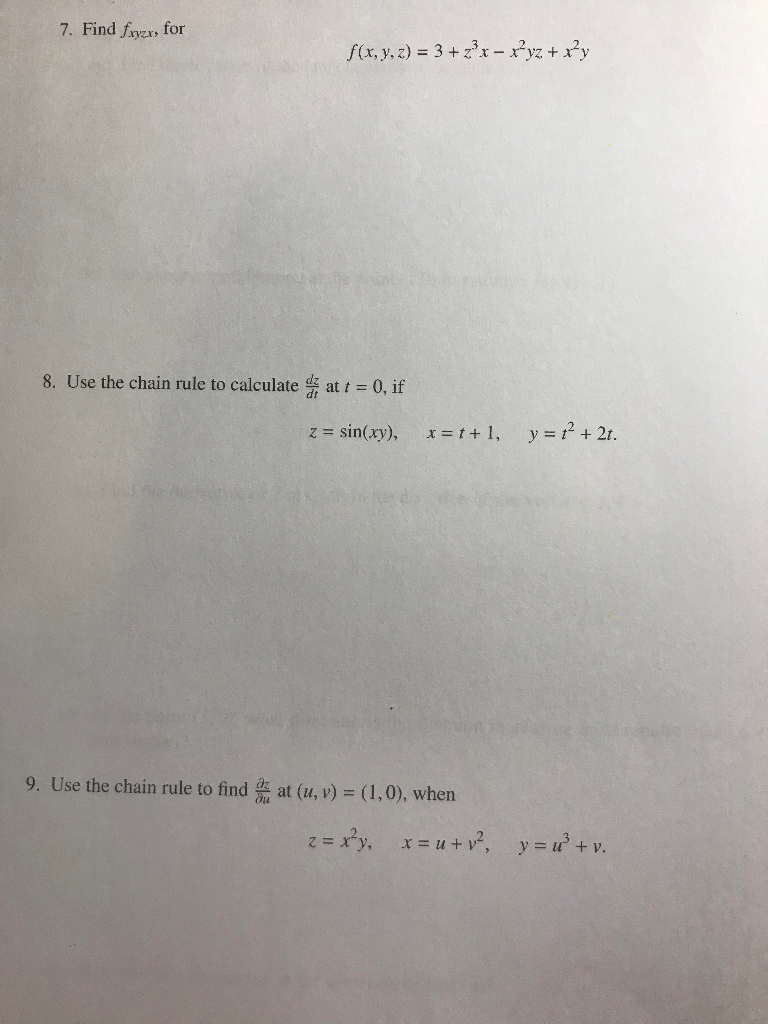please help with these questions 7. Find fryzx, for f(x, y, z) = 3 + 2?x – xyz + x+y 8. Use the chain rule to calculate that t = 0, if z = sin(xy), x = 1+1, y = 12 + 2t. 9. Use the chain rule to find us at (u, v) = (1,0), when z = xy, x = u +v?, y = x + v.

• ### (1 point) Use the chain rule to find ow, where u0 = xy + yz,x =...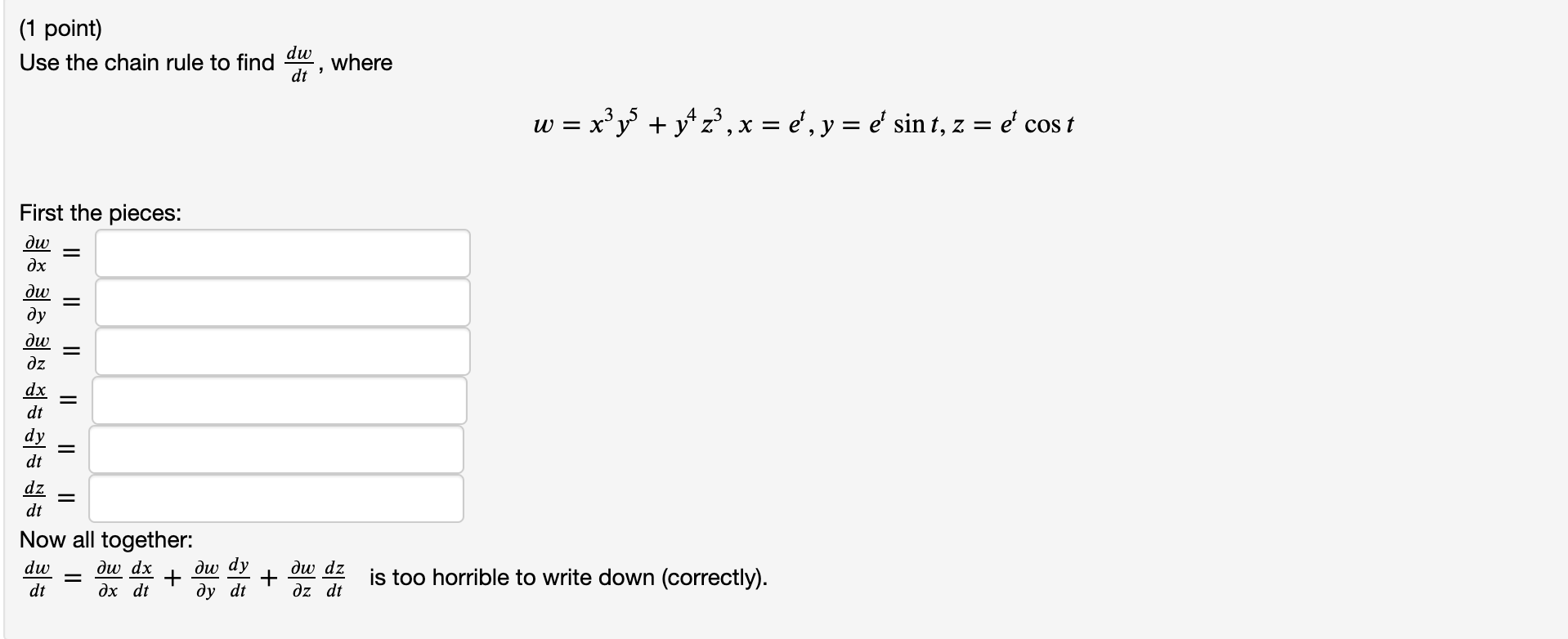(1 point) Use the chain rule to find ow, where u0 = xy + yz,x = d, y = e sin t, z = e cost First the pieces: Now all together: dw = dw dx + dw dy + dw dz is too horrible to di – og det du di + Öz do is too horrible to write down (correctly).

• ### what is the answer for number 4 1. Let r(t) = -i-e2t j + (t? +...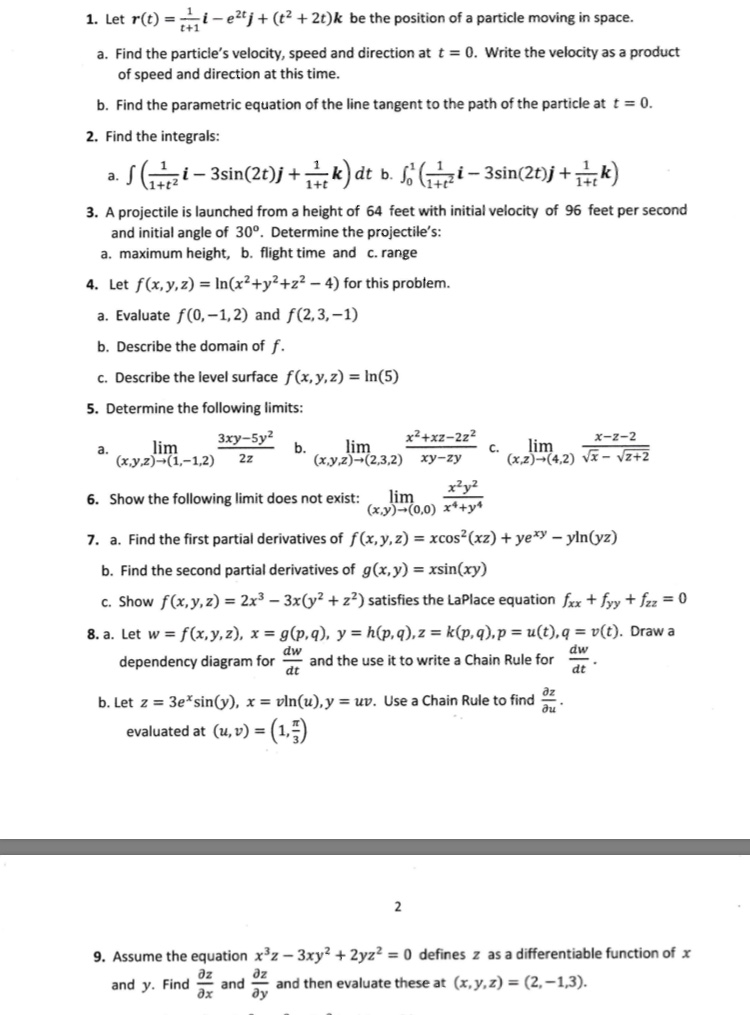what is the answer for number 4 1. Let r(t) = -i-e2t j + (t? + 2t)k be the position of a particle moving in space. a. Find the particle's velocity, speed and direction at t = 0. Write the velocity as a product of speed and direction at this time. b. Find the parametric equation of the line tangent to the path of the particle at t = 0. 2. Find the integrals: a. S (tezi - 3sin(2t)j +...